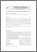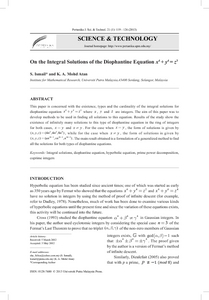# On the integral solutions of the diophantine equation x4 + y4 = z3

## Citation

Ismail, S. and Mohd Atan, Kamel Ariffin (2013) On the integral solutions of the diophantine equation x4 + y4 = z3. Pertanika Journal of Science & Technology, 21 (1). pp. 119-126. ISSN 0128-7680; ESSN: 2231-8526

## Abstract

This paper is concerned with the existence, types and the cardinality of the integral solutions for diophantine equation x4y4z3+ = where x , y and z are integers. The aim of this paper was to develop methods to be used in finding all solutions to this equation. Results of the study show the existence of infinitely many solutions to this type of diophantine equation in the ring of integers for both cases, x=y and x y. For the case when x=y, the form of solutions is given by (x,y,z)=(4n3,4n3,8n4), while for the case when x y, the form of solutions is given by (x,y,z)=(un3k-1,vn3k-1,n4k-1). The main result obtained is a formulation of a generalized method to find all the solutions for both types of diophantine equations.Preview
PDF
On the Integral Solutions of the Diophantine Equation x4 + y4 = z³4.pdfView Item